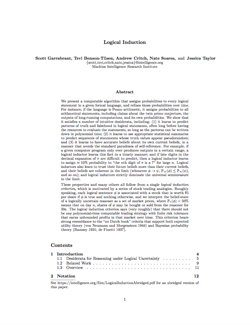Logical Induction

This is a preprint of a new paper on reasoning under deductive limitations, co-authored by Scott Garrabrant, Tsvi Benson-Tilsen, Andrew Critch, Nate Soares, and Jessica Taylor.

Logical Induction — PreprintAbstract: We present a computable algorithm that assigns probabilities to every logical statement in a given formal language, and refines those probabilities over time. For instance, if the language is Peano arithmetic, it assigns probabilities to all arithmetical statements, including claims about the twin prime conjecture, the outputs of long-running computations, and its own probabilities.

We show that our algorithm, an instance of what we call a logical inductor, satisfies a number of intuitive desiderata, including: (1) it learns to predict patterns of truth and falsehood in logical statements, often long before having the resources to evaluate the statements, so long as the patterns can be written down in polynomial time; (2) it learns to use appropriate statistical summaries to predict sequences of statements whose truth values appear pseudorandom; and (3) it learns to have accurate beliefs about its own current beliefs, in a manner that avoids the standard paradoxes of self-reference. For example, if a given computer program only ever produces outputs in a certain range, a logical inductor learns this fact in a timely manner; and if late digits in the decimal expansion of π are difficult to predict, then a logical inductor learns to assign ≈ 10% probability to “the nth digit of π is a 7” for large n. Logical inductors also learn to trust their future beliefs more than their current beliefs, and their beliefs are coherent in the limit (whenever φψ, ℙ(φ) ≤ ℙ(ψ), and so on); and logical inductors strictly dominate the universal semimeasure in the limit.

These properties and many others all follow from a single logical induction criterion, which is motivated by a series of stock trading analogies. Roughly speaking, each logical sentence φ is associated with a stock that is worth \$1 per share if φ is true and nothing otherwise, and we interpret the belief-state of a logically uncertain reasoner as a set of market prices, where Pn(φ) = 50% means that on day n, shares of φ may be bought or sold from the reasoner for 50¢. The logical induction criterion says (very roughly) that there should not be any polynomial-time computable trading strategy with finite risk tolerance that earns unbounded profits in that market over time. This criterion bears strong resemblance to the “no Dutch book” criteria that support both expected utility theory (Von-Neumann, 19441) and Bayesian probability theory (Ramsey, 1931;2 de Finetti, 19373).

Logical Induction — Talk

Andrew Critch discusses some properties of logical inductors. See here for a longer version of the same talk, with motivations and directions for future research.

1. Von Neumann, John, and Oskar Morgenstern. 1944. Theory of Games and Economic Behavior. 1st ed. Princeton, NJ: Princeton University Press. []
2. Ramsey, Frank Plumpton. 1931. “Truth and Probability.” In The Foundations of Mathematics and other Logical Essays, edited by Richard Bevan Braithwaite, 156–198. New York: Harcourt, Brace. []
3. de Finetti, Bruno. 1937. “Foresight: Its Logical Laws, Its Subjective Sources.” In Studies in Subjective Probability, edited by Henry E. Kyburg and Howard E.K. Smokler. Huntington, New York: Roger E. Kreiger Publishing Co. []